# Excel LEFT function with formula examples

The tutorial shows how to use the LEFT function in Excel to get a substring from the beginning of the text string, extract text before a certain character, force a Left formula to return a number, and more.

Among many different functions that Microsoft Excel provides for manipulating text data, LEFT is one of the most widely used. As its name suggests, the function allows you to extract a certain number of characters starting at the left side of a text string. However, Excel LEFT is capable of much more than its pure essence. In this tutorial, you will find a couple of basic Left formulas to understand the syntax, and then I will show you a few ways in which you can take the Excel LEFT function well beyond its basic usage.

## Excel LEFT function - syntax

The LEFT function in Excel returns the specified number of characters (substring) from the start of a string.

The syntax of the LEFT function is as follows:

LEFT(text, [num_chars])

Where:

• Text (required) is the text string from which you want to extract a substring. Usually it is supplied as a reference to the cell containing the text.
• Num_chars (optional) - the number of characters to extract, starting on the left side of the string.
• If num_chars is omitted, it defaults to 1, meaning that a Left formula will return 1 character.
• If num_chars is greater than the total length of text, a Left formula will return all of text.

For example, to extract the first 3 characters from the text in cell A2, use this formula:

`=LEFT(A2, 3)`

The following screenshot shows the result: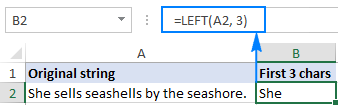Important note! LEFT belongs to the category of Text functions, therefore the result of a Left formula is always a text string, even if the original value from which you extract characters is a number. If you are working with a numeric dataset and want the LEFT function to return a number, use it in conjunction with the VALUE function as demonstrated in this example.

## How to use LEFT function in Excel - formula examples

Apart from extracting text from the left of a string, what else can the LEFT function do? The following examples show how you can use LEFT in combination with other Excel functions to solve more complex tasks.

### How to extract a substring before a certain character

In some cases, you may need to extract the part of the text string that precedes a specific character. For example, you may want to pull the first names from a column of full names or get the country codes from a column of phone numbers. The problem is that each name and each code contains a different number of characters, and therefore you cannot simply supply a predefined number to the num_chars argument of your Left formula like we did in the above example.

If the first and last names are separated by a space, the problem boils down to working out the position of the space character in a string, which can be easily done by using either SEARCH or FIND function.

Supposing the full name is in cell A2, the position of the space is returned by this simple formula: SEARCH(" ",A2)). And now, you embed this formula in the num_chars argument of the LEFT function:

`=LEFT(A2, SEARCH(" ", A2))`

To improve the formula a bit further, get rid of the trailing space by subtracting 1 from the Search formula result (not visible in cells, trailing spaces may cause many problems especially if you plan to use the extracted names in other formulas):

`=LEFT(A2, SEARCH(" ", A2)-1)`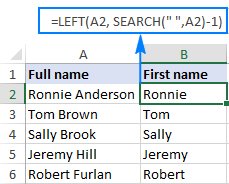In the same fashion, you can extract the country codes from a column of telephone numbers. The only difference is that you use the Search function to find out the position of the first hyphen ("-") rather than a space:

`=LEFT(A2, SEARCH("-", A2)-1)`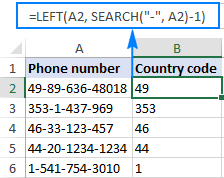Wrapping up, you can use this generic formula to get a substring that precedes any other character:

LEFT(string, SEARCH(character, string) - 1)

### How to remove the last N characters from a string

You already know how to use the Excel LEFT function to get a substring from the start of a text string. But sometimes you may want to do something different - remove a certain number of characters from the end of the string and pull the rest of the string into another cell. For this, use the LEFT function in combination with LEN, like this:

LEFT(string, LEN(string) - number_of_chars_to_remove)

The formula works with this logic: the LEN function gets the total number of characters in a string, then you subtract the number of unwanted characters from the total length, and have the LEFT function return the remaining characters.

For example, to remove the last 7 characters from text in A2, use this formula:

`=LEFT(A2, LEN(A2)-7)`

As shown in the screenshot below, the formula successfully cuts off the " - ToDo" postfix (4 letters, a hyphen and 2 spaces) from the text strings in column A.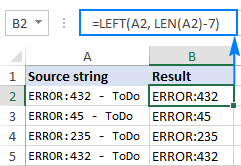### How to force the LEFT function to return a number

As you already know, the Excel LEFT function always returns text, even when you are pulling a few first digits from a number. What it means to you is that you won't be able to use the results of your Left formulas in calculations or in other Excel functions that operate on numbers.

So, how do you make Excel LEFT to output a number rather than a text string? Simply by wrapping it in the VALUE function, which is designed to convert a string representing a number to a number, like this: `VALUE(LEFT())`

For example, to extract the first 2 characters from the string in A2 and convert the output into numbers, use this formula:

`=VALUE(LEFT(A2,2))`

The result will look something similar to this: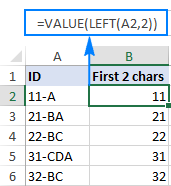As you can see in the screenshot above, the numbers in column B obtained with a Value Left formula are right-alighted in cells, as opposed to left-aligned text in column A. Since Excel recognizes the output as numbers, you are free to sum and average those values, find the min and max value, and perform any other calculations.

These are just a few of many possible uses of LEFT in Excel. To have a closer look at the formulas discussed in this tutorial, you are welcome to download the Excel LEFT function sample worksheet.

For more Left formula examples, please check out the following resources:

## Excel LEFT function not working - reasons and solutions

If the Excel LEFT function does not work properly in your worksheets, it's most likely because of one of the following reasons.

### 1. Num_chars argument is less than zero

If your Excel Left formula returns the #VALUE! error, the first thing for you to check is the value in the num_chars argument. If it's a negative number, just remove the minus sign and the error will be gone (of course, it's very unlikely that someone will put a negative number there of purpose, but to err is human :)

Most often, the VALUE error occurs when the num_chars argument is represented by another function. In this case, copy that function to another cell or select it in the formula bar and press F9 to see what it equates to. If the value is less than 0, then check the function for errors.

To better illustrate the point, let's take the Left formula we've used in the first example to extract the country phone codes: LEFT(A2, SEARCH("-", A2)-1). As you may remember, the Search function in the num_chars argument calculates the position of the first hyphen in the original string, from which we subtract 1 to remove the hyphen from the final result. If I accidentally replace -1, say, with -11, the formula would through the #VALUE error because the num_chars argument equates to a negative numbers: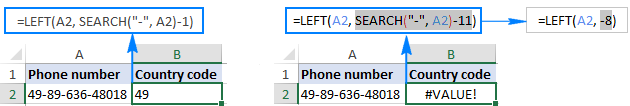### 2. Leading spaces in the original text

In case your Excel Left formula fails for no obvious reason, check the original values for leading spaces. If you have copied your data from the web or exported from another external source, many such spaces may lurk unnoticed before the text entries, and you will never know they are there until something goes wrong. The following image illustrates the problem: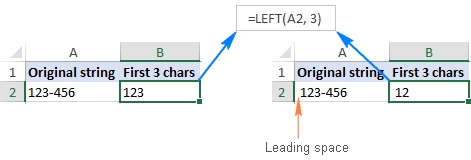To get rid of the leading spaces in your worksheets, use the Excel TRIM function or the Text Toolkit add-in.

### 3. Excel LEFT does not work with dates

If you attempt use the Excel LEFT function to get an individual part of a date (such as day, month or year), in most cases you will only retrieve the first few digits of the number that represents that date. The point is that in Microsoft Excel, all dates are stored as integers representing the number of days since January 1, 1900, which is stored as number 1 (for more information, please see Excel date format). What you see in a cell is just a visual representation of the date and its display can easily be changed by applying a different date format.

For example, if you have the date 11-Jan-2017 in cell A1 and you try to extract the day by using the formula LEFT(A1,2), the result would be 42, which is the first 2 digits of number 42746 that represents January 11, 2017 in the internal Excel system.

To extract a specific part of a date, use one of the following functions: DAY, MONTH or YEAR.

In case your dates are entered as text strings, the LEFT function will work without a hitch, as shown in the right part of the screenshot: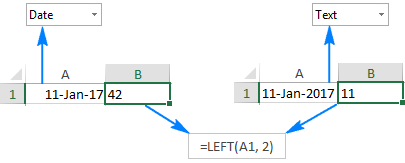This is how you use the LEFT function in Excel. I thank you for reading and hope to see you again next week.

## You may also be interested in

1. I am trying to separate a percent number from a course in a cell, when there are multiple terms.
For example Cells may be
88%- ENGL100, 100%- MATH111
92%- BUSN115, 75%- ENGL100
72%- ENGL100

I only was to see the percent for one class, so I can then create an if statement to find the letter grade.
How can I return only the 4 characters, or numbers before ENGL100- if the order of the classes may differ from cell to cell?

2. given date in Excel sheet like this column format
for example
MP TITLE unit_count.type unit_count package Expire category comment pack size batch
111172 Mornington Peninsula Brewery IPA (Can) 330mL Case of 24 millilitre 7920 Y Y Beer Case of 24 Batch- 5
111172 Mornington Peninsula Brewery Lager (Can) 330mL Case Of 24 millilitre 7920 Y Y Beer Case of 24 Batch- 5
111172 Mornington Peninsula Brewery Pale (Can) 330mL Case Of 24 millilitre 7920 Y Y Beer Case of 24 Batch- 5
111172 Mornington Peninsula Brewery Pale 375mL Case of 24 Liters 0.375 Y Y Beer case of 24 Batch- 5
111172 Mornington Peninsula Brewery The Continuous Daryl 330mL Case Of 24 millilitre 7920 Y Y Beer Pack of 24 Batch- 5
111172 Mornington Peninsula Brewery VinAle Red 750mL Case of 6 Liters 0.75 N Y Beer Case of 6 Batch- 5
111172 Mornington Peninsula Brewery XPA 375mL Case of 24 Liters 0.375 Y Y Beer Case of 24 Batch- 5
111172 Morris Bin 158 Durif Red Wine 750 ml millilitre 750 N N Wine Single Pack Batch- 6
111172 Morris Classic Muscat Liqueur 750 ml millilitre 750 N N Spirit Single Pack Batch- 6
111172 Morris Kitchen - Tequila Mixer Set - 16oz (2 pk) Other Non Alchole Product Batch- 6
111172 Morris Rutherglen Muscat Barrel Finished Single Malt Australian Whisky 700ml millilitre 700 N N Spirit Single Pack Batch- 6
111172 Morris Rutherglen Signature Single Malt Australian Whisky 700ml millilitre 700 N N Spirit Single Pack. Batch- 6
111172 Morris Sparkling Shiraz Durif Red Wine 750 ml millilitre 750 N N Wine Single Pack Batch- 6
111172 Morris Wines Classic Liqueur Muscat 50 cl (Case of 6), 500 ml (Pack Of 6) millilitre 3000 N N Spirit Case of 6 Batch- 5

but I wanted to data in Sheet 2 column format
TITLE category MP package Expire unit_count unit_count.type pack size batch comment

• I just wanted to rearrange data automatically Sheet 1 to sheet2
is it possible?

• Hello!
You can move columns manually. The fastest way is to use the special Column Manager tool. It is available as a part of our Ultimate Suite for Excel that you can install in a trial mode and check how it works for free.
You can also use the new Excel function CHOOSECOLS. You can find detailed instructions and examples in this article: CHOOSECOLS function in Excel to get columns from array or range